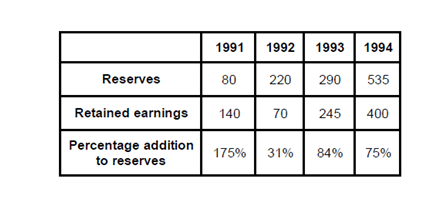Direction for questions 1 to 4: Answer the questions based on the following information.
Ramesh surveyed his companies and obtained the following data. Income tax is paid from profit before tax and the remaining amount is distributed to dividend and retained earnings. The retained earnings were gathered into reserves. The reserves at the beginning of 1991 were Rs. 80 lakh.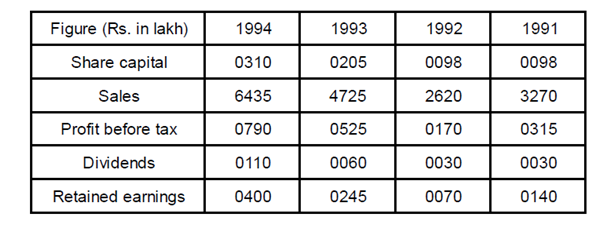Question 1.  After Ramesh surveyed his companies, in which year did he find the tax per rupee of profit before tax’ to be the lowest?
a. 1991
b. 1992
c. 1993
d. 1994

Question 2. In which year was the sales per rupee of share capital highest?
a. 1991
b. 1992
c. 1993
d. 1994

Question 3. In which year the profit before tax per rupee of sales was the highest?
a. 1991
b. 1992
c. 1993
d. 1994

Question 4. In which year did Ramesh find that the percentage addition to reserves over previous years reserves the highest?
a. 1991
b. 1992
c. 1993
d. 1994

Question 5. Amount of the reserves at the end of 1993 is
a. Rs. 535 lakh
b. Rs. 515 lakh
c. Rs. 230 lakh
d. None of these

We know that (Profit before tax) – Tax = Dividends + Retained earnings

∴Tax = (Profit before tax) – (Dividends + Retainedearnings)

From the given table we can calculate the tax for each year as follows: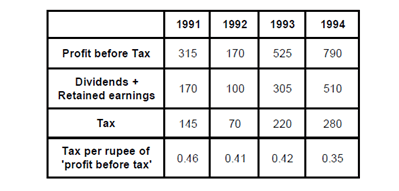Hence, from above table, Tax per rupee of ‘profit before tax’ is lowest for 1994.

Sales per rupee of share capital = Sales / Share capital

We have calculated the same for each year below: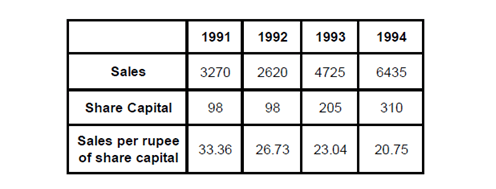From the table we can say that sales per rupee of the share capital is highest for 1991 i.e. 33.36

Profit before tax per rupee of sales = profit before tax / sales

The table below shows the calculations for each year: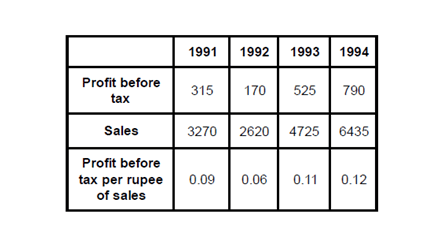Hence, profit before tax per rupee of sales is highest for 1994.

Percentage addition to reserves = Retained earnings / Reserves  ×100